# 10.6 Introduction to factoring polynomials  (Page 2/6)

 Page 2 / 6

Find the greatest common factor: $16{x}^{2},\phantom{\rule{0.2em}{0ex}}24{x}^{3}.$

8 x 2

Find the greatest common factor: $27{y}^{3},\phantom{\rule{0.2em}{0ex}}18{y}^{4}.$

9 y 3

Find the greatest common factor of $14{x}^{3},\phantom{\rule{0.2em}{0ex}}8{x}^{2},\phantom{\rule{0.2em}{0ex}}10x.$

## Solution

 Factor each coefficient into primes and write the variables with exponents in expanded form. Circle the common factors in each column. Bring down the common factors. Multiply the factors.$\text{The GCF of}\phantom{\rule{0.2em}{0ex}}14{x}^{3}\phantom{\rule{0.2em}{0ex}}\text{and}\phantom{\rule{0.2em}{0ex}}8{x}^{2}\text{, and}10x\phantom{\rule{0.2em}{0ex}}\text{is}\phantom{\rule{0.2em}{0ex}}2x$

Find the greatest common factor: $21{x}^{3},\phantom{\rule{0.2em}{0ex}}9{x}^{2},\phantom{\rule{0.2em}{0ex}}15x.$

3 x

Find the greatest common factor: $25{m}^{4},\phantom{\rule{0.2em}{0ex}}35{m}^{3},\phantom{\rule{0.2em}{0ex}}20{m}^{2}.$

5 m 2

## Factor the greatest common factor from a polynomial

Just like in arithmetic, where it is sometimes useful to represent a number in factored form (for example, $12$ as $2·6\phantom{\rule{0.2em}{0ex}}\text{or}\phantom{\rule{0.2em}{0ex}}3·4\text{),}$ in algebra it can be useful to represent a polynomial in factored form. One way to do this is by finding the greatest common factor of all the terms. Remember that you can multiply a polynomial by a monomial as follows:

$\begin{array}{ccc}\hfill 2\left(x& +& 7\right)\phantom{\rule{0.2em}{0ex}}\text{factors}\hfill \\ \hfill 2·x& +& 2·7\hfill \\ \hfill 2x& +& 14\phantom{\rule{0.2em}{0ex}}\text{product}\hfill \end{array}$

Here, we will start with a product, like $2x+14,$ and end with its factors, $2\left(x+7\right).$ To do this we apply the Distributive Property “in reverse”.

## Distributive property

If $a,\phantom{\rule{0.2em}{0ex}}b,\phantom{\rule{0.2em}{0ex}}c$ are real numbers, then

$a\left(b+c\right)=ab+ac\phantom{\rule{2em}{0ex}}\text{and}\phantom{\rule{2em}{0ex}}ab+ac=a\left(b+c\right)$

The form on the left is used to multiply. The form on the right is used to factor.

So how do we use the Distributive Property to factor a polynomial? We find the GCF of all the terms and write the polynomial as a product!

Factor: $2x+14.$

## Solution

 Step 1: Find the GCF of all the terms of the polynomial. Find the GCF of 2x and 14.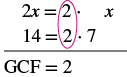Step 2: Rewrite each term as a product using the GCF. Rewrite 2x and 14 as products of their GCF, 2. $2x=2\cdot x$ $14=2\cdot 7$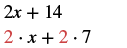Step 3: Use the Distributive Property 'in reverse' to factor the expression. $2\left(x+7\right)$ Step 4: Check by multiplying the factors. Check: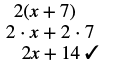Factor: $4x+12.$

4( x + 3)

Factor: $6a+24.$

6( a + 4)

Notice that in [link] , we used the word factor as both a noun and a verb:

$\begin{array}{cccc}\text{Noun}\hfill & & & 7\phantom{\rule{0.2em}{0ex}}\text{is a factor of}\phantom{\rule{0.2em}{0ex}}14\hfill \\ \text{Verb}\hfill & & & \text{factor}\phantom{\rule{0.2em}{0ex}}2\phantom{\rule{0.2em}{0ex}}\text{from}\phantom{\rule{0.2em}{0ex}}2x+14\hfill \end{array}$

## Factor the greatest common factor from a polynomial.

1. Find the GCF of all the terms of the polynomial.
2. Rewrite each term as a product using the GCF.
3. Use the Distributive Property ‘in reverse’ to factor the expression.
4. Check by multiplying the factors.

Factor: $3a+3.$

## Solution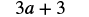Rewrite each term as a product using the GCF.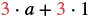Use the Distributive Property 'in reverse' to factor the GCF.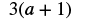Check by multiplying the factors to get the original polynomial.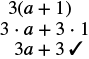Factor: $9a+9.$

9( a + 1)

Factor: $11x+11.$

11( x + 1)

The expressions in the next example have several factors in common. Remember to write the GCF as the product of all the common factors.

Factor: $12x-60.$

## Solution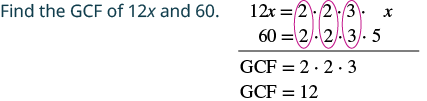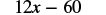Rewrite each term as a product using the GCF.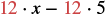Factor the GCF.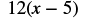Check by multiplying the factors.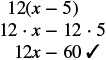Factor: $11x-44.$

11( x − 4)

Factor: $13y-52.$

13( y − 4)

Now we’ll factor the greatest common factor    from a trinomial    . We start by finding the GCF of all three terms.

Factor: $3{y}^{2}+6y+9.$

## Solution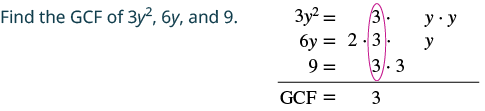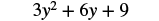Rewrite each term as a product using the GCF.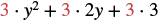Factor the GCF.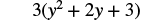Check by multiplying.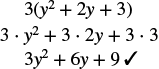Factor: $4{y}^{2}+8y+12.$

4( y 2 + 2 y + 3)

Factor: $6{x}^{2}+42x-12.$

6( x 2 + 7 x − 2)

In the next example, we factor a variable from a binomial    .

Factor: $6{x}^{2}+5x.$

## Solution

 $6{x}^{2}+5x$ Rewrite each term as a product.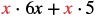Factor the GCF. $x\left(6x+5\right)$ Check by multiplying. $x\left(6x+5\right)$ $x\cdot 6x+x\cdot 5$ $6{x}^{2}+5x✓$

#### Questions & Answers

show that the set of all natural number form semi group under the composition of addition
Nikhil Reply
what is the meaning
Dominic
explain and give four Example hyperbolic function
Lukman Reply
_3_2_1
felecia
⅗ ⅔½
felecia
_½+⅔-¾
felecia
The denominator of a certain fraction is 9 more than the numerator. If 6 is added to both terms of the fraction, the value of the fraction becomes 2/3. Find the original fraction. 2. The sum of the least and greatest of 3 consecutive integers is 60. What are the valu
SABAL Reply
1. x + 6 2 -------------- = _ x + 9 + 6 3 x + 6 3 ----------- x -- (cross multiply) x + 15 2 3(x + 6) = 2(x + 15) 3x + 18 = 2x + 30 (-2x from both) x + 18 = 30 (-18 from both) x = 12 Test: 12 + 6 18 2 -------------- = --- = --- 12 + 9 + 6 27 3
Pawel
2. (x) + (x + 2) = 60 2x + 2 = 60 2x = 58 x = 29 29, 30, & 31
Pawel
ok
Ifeanyi
on number 2 question How did you got 2x +2
Ifeanyi
combine like terms. x + x + 2 is same as 2x + 2
Pawel
x*x=2
felecia
2+2x=
felecia
×/×+9+6/1
Debbie
Q2 x+(x+2)+(x+4)=60 3x+6=60 3x+6-6=60-6 3x=54 3x/3=54/3 x=18 :. The numbers are 18,20 and 22
Naagmenkoma
Mark and Don are planning to sell each of their marble collections at a garage sale. If Don has 1 more than 3 times the number of marbles Mark has, how many does each boy have to sell if the total number of marbles is 113?
mariel Reply
Mark = x,. Don = 3x + 1 x + 3x + 1 = 113 4x = 112, x = 28 Mark = 28, Don = 85, 28 + 85 = 113
Pawel
how do I set up the problem?
Harshika Reply
what is a solution set?
Harshika
find the subring of gaussian integers?
Rofiqul
hello, I am happy to help!
Shirley Reply
please can go further on polynomials quadratic
Abdullahi
hi mam
Mark
I need quadratic equation link to Alpa Beta
Abdullahi Reply
find the value of 2x=32
Felix Reply
divide by 2 on each side of the equal sign to solve for x
corri
X=16
Michael
Want to review on complex number 1.What are complex number 2.How to solve complex number problems.
Beyan
yes i wantt to review
Mark
16
Makan
x=16
Makan
use the y -intercept and slope to sketch the graph of the equation y=6x
Only Reply
how do we prove the quadratic formular
Seidu Reply
please help me prove quadratic formula
Darius
hello, if you have a question about Algebra 2. I may be able to help. I am an Algebra 2 Teacher
Shirley Reply
thank you help me with how to prove the quadratic equation
Seidu
may God blessed u for that. Please I want u to help me in sets.
Opoku
what is math number
Tric Reply
4
Trista
x-2y+3z=-3 2x-y+z=7 -x+3y-z=6
Sidiki Reply
can you teacch how to solve that🙏
Mark
Solve for the first variable in one of the equations, then substitute the result into the other equation. Point For: (6111,4111,−411)(6111,4111,-411) Equation Form: x=6111,y=4111,z=−411x=6111,y=4111,z=-411
Brenna
(61/11,41/11,−4/11)
Brenna
x=61/11 y=41/11 z=−4/11 x=61/11 y=41/11 z=-4/11
Brenna
Need help solving this problem (2/7)^-2
Simone Reply
x+2y-z=7
Sidiki
what is the coefficient of -4×
Mehri Reply
-1
Shedrak
A soccer field is a rectangle 130 meters wide and 110 meters long. The coach asks players to run from one corner to the other corner diagonally across. What is that distance, to the nearest tenths place.
Kimberly Reply
Jeannette has $5 and$10 bills in her wallet. The number of fives is three more than six times the number of tens. Let t represent the number of tens. Write an expression for the number of fives.
August Reply
What is the expressiin for seven less than four times the number of nickels
Leonardo Reply
How do i figure this problem out.
how do you translate this in Algebraic Expressions
linda Reply
why surface tension is zero at critical temperature
Shanjida
I think if critical temperature denote high temperature then a liquid stats boils that time the water stats to evaporate so some moles of h2o to up and due to high temp the bonding break they have low density so it can be a reason
s.
Need to simplify the expresin. 3/7 (x+y)-1/7 (x-1)=
Crystal Reply
. After 3 months on a diet, Lisa had lost 12% of her original weight. She lost 21 pounds. What was Lisa's original weight?
Chris Reply

### Read also:

#### Get Jobilize Job Search Mobile App in your pocket Now!

Source:  OpenStax, Prealgebra. OpenStax CNX. Jul 15, 2016 Download for free at http://legacy.cnx.org/content/col11756/1.9
Google Play and the Google Play logo are trademarks of Google Inc.

Notification Switch

Would you like to follow the 'Prealgebra' conversation and receive update notifications?By OpenStaxBy Vongkol HENGBy Brooke DelaneyBy Candice ButtsBy JavaChamp TeamBy Angelica LitoBy OpenStaxBy Dakota BocanBy OpenStaxBy Richley Crapo